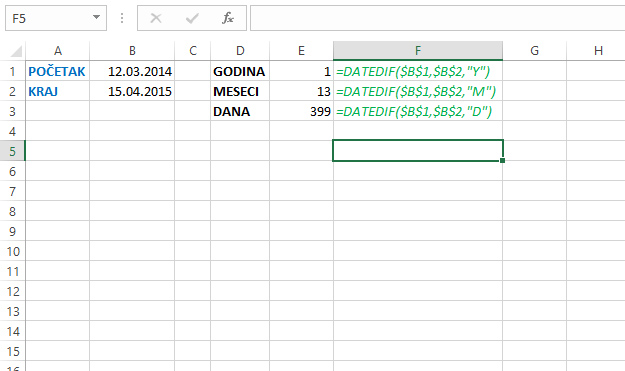Difference in dates

In one of the previous “recipes” you could find out how to calculate the number of past days between two dates. Excel has another function that can be used for this purpose. It is a hidden DATEDIF function, which, for two given dates, can calculate the number of years, months or days. The following text will be about how this function is used …

DATEDIF function syntax is je:

DATEDIF (<start date>,<end date>,<interval>)

The first two arguments of this function are the dates between which we count the past number of periods (year, month or day). The third argument is the letter “Y”, “M” or “D” that indicates years, months or days. For example, if we enter the formula:

=DATEDIF(\$B\$1,\$B\$2,”M”)

we will get between 13 months, between the two given dates, in cells B1 and B2.